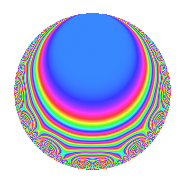# Properties

 Label 123.2.dLevel 123 Weight 2 Character orbit d Rep. character $$\chi_{123}(40,\cdot)$$ Character field $$\Q$$ Dimension 8 Newforms 1 Sturm bound 28 Trace bound 0

# Related objects

## Defining parameters

 Level: $$N$$ = $$123 = 3 \cdot 41$$ Weight: $$k$$ = $$2$$ Character orbit: $$[\chi]$$ = 123.d (of order $$2$$ and degree $$1$$) Character conductor: $$\operatorname{cond}(\chi)$$ = $$41$$ Character field: $$\Q$$ Newforms: $$1$$ Sturm bound: $$28$$ Trace bound: $$0$$

## Dimensions

The following table gives the dimensions of various subspaces of $$M_{2}(123, [\chi])$$.

Total New Old
Modular forms 16 8 8
Cusp forms 12 8 4
Eisenstein series 4 0 4

## Trace form

 $$8q + 2q^{2} + 10q^{4} - 8q^{5} - 6q^{8} - 8q^{9} + O(q^{10})$$ $$8q + 2q^{2} + 10q^{4} - 8q^{5} - 6q^{8} - 8q^{9} - 4q^{10} + 6q^{16} - 2q^{18} - 8q^{20} - 4q^{21} - 4q^{23} + 8q^{25} + 26q^{31} - 10q^{32} - 10q^{33} - 10q^{36} + 10q^{37} - 8q^{39} - 36q^{40} + 30q^{41} + 20q^{42} - 2q^{43} + 8q^{45} - 8q^{46} - 8q^{49} + 34q^{50} - 6q^{51} + 12q^{57} - 24q^{59} + 6q^{61} - 40q^{62} - 42q^{64} + 6q^{72} - 18q^{73} + 100q^{74} + 36q^{77} + 28q^{78} - 44q^{80} + 8q^{81} - 18q^{82} + 36q^{84} - 28q^{86} - 26q^{87} + 4q^{90} + 16q^{91} + 84q^{92} - 38q^{98} + O(q^{100})$$

## Decomposition of $$S_{2}^{\mathrm{new}}(123, [\chi])$$ into irreducible Hecke orbits

Label Dim. $$A$$ Field CM Traces $q$-expansion
$$a_2$$ $$a_3$$ $$a_5$$ $$a_7$$
123.2.d.a $$8$$ $$0.982$$ $$\mathbb{Q}[x]/(x^{8} + \cdots)$$ None $$2$$ $$0$$ $$-8$$ $$0$$ $$q+\beta _{4}q^{2}+\beta _{3}q^{3}+(1-\beta _{2})q^{4}+(-1+\cdots)q^{5}+\cdots$$

## Decomposition of $$S_{2}^{\mathrm{old}}(123, [\chi])$$ into lower level spaces

$$S_{2}^{\mathrm{old}}(123, [\chi]) \cong$$ $$S_{2}^{\mathrm{new}}(41, [\chi])$$$$^{\oplus 2}$$